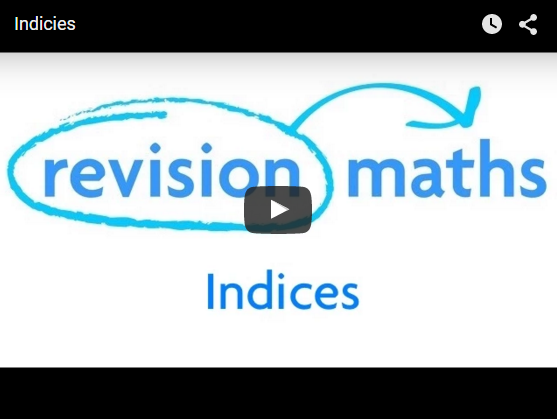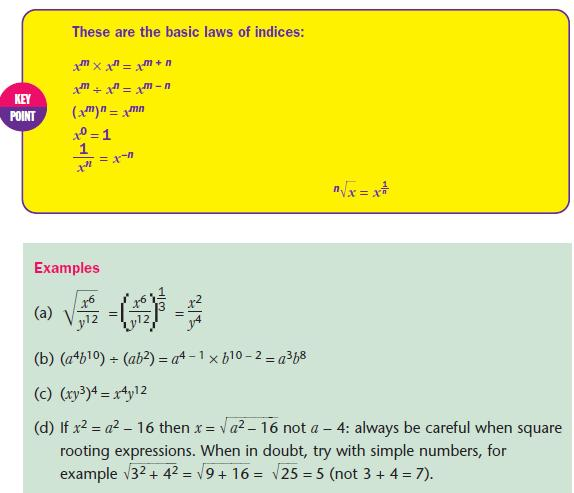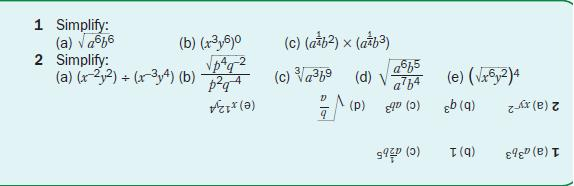## Indices

This section covers Indices and the uses of Indices in algebra. After studying this section, you will be able to:

• divide and multiply algebraic expressions using indices
• find roots using indices.

This video shows a guide to indices and powers. Multiplying and dividing indices, raising indices to a power and using standard form are explained.Using the rules of indices